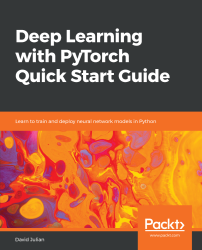•#### Deep Learning with PyTorch Quick Start Guide#### Overview of this book

PyTorch is extremely powerful and yet easy to learn. It provides advanced features, such as supporting multiprocessor, distributed, and parallel computation. This book is an excellent entry point for those wanting to explore deep learning with PyTorch to harness its power. This book will introduce you to the PyTorch deep learning library and teach you how to train deep learning models without any hassle. We will set up the deep learning environment using PyTorch, and then train and deploy different types of deep learning models, such as CNN, RNN, and autoencoders. You will learn how to optimize models by tuning hyperparameters and how to use PyTorch in multiprocessor and distributed environments. We will discuss long short-term memory network (LSTMs) and build a language model to predict text. By the end of this book, you will be familiar with PyTorch's capabilities and be able to utilize the library to train your neural networks with relative ease.
PrefaceFree Chapter
Introduction to PyTorchDeep Learning FundamentalsComputational Graphs and Linear ModelsConvolutional NetworksOther NN ArchitecturesGetting the Most out of PyTorchOther Books You May Enjoy# Getting the Most out of PyTorch

By now, you should be able to build and train three different types of model: linear, convolutional, and recurrent. You should have an appreciation of the theory and mathematics behind these model architectures and explain how they make predictions. Convolutional networks are probably the most studied deep learning network, especially in relation to image data. Of course, both convolutional and recurrent networks make extensive use of linear layers, so the theory behind linear networks, most notably linear regression and gradient descent, is fundamental to all artificial neural networks.

Our discussion so far has been fairly contained. We have looked at a well-studied problem, such as classification using MNIST, to give you a solid understanding of the basic PyTorch building blocks. This final chapter is the launching pad for your use of PyTorch...